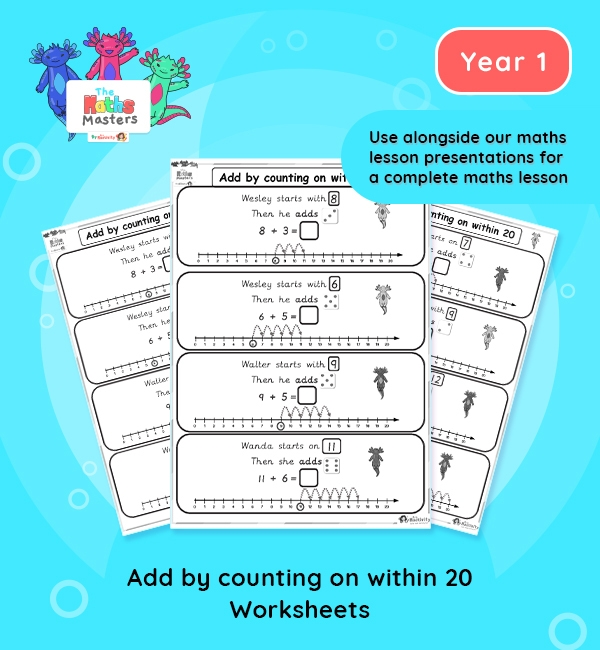# Year 1 | Adding to 20 by Counting on Worksheets## Year 1 addition and subtraction worksheets

Aligned with the maths mastery approach, this Year 1 | Adding to 20 by Counting on Worksheets are designed to be used alongside our Year 1 | Adding to 20 by Counting on Lesson Presentation Lesson Presentation to provide a complete maths lesson. These worksheets require children to use their knowledge of counting on to solve addition problems.

Small Steps: Add by counting on (within 20).

NC Links: * Read, write and interpret mathematical statements involving addition, subtraction and equals signs. *Add and subtract one-digit and two-digit numbers to 20, including zero.

TAF Statements: Working towards: Add and subtract (one digit numbers) explaining their method verbally, in pictures, or using apparatus.

Greater Depth – Use reasoning about number and relationships to solve more complex problems and explain their thinking.

Ready-to-progress criteria:  1NF-1 Develop fluency in addition and subtraction facts within 10

Future applications: Add and subtract across 10.

1AS-2 Read, write and interpret equations containing addition(+), subtraction (-), and equals (=) symbols, and relate additive expressions and equations to real-life contexts.

Previous experience: Devise and record number stories, using pictures, numbers and symbols.

## Recently Viewed